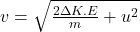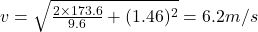## A crate of mass 9.6 kg is pulled up a rough incline with an initial speed of 1.46 m/s. The pulling force is 102 N parallel to the incline, w

Question

A crate of mass 9.6 kg is pulled up a rough incline with an initial speed of 1.46 m/s. The pulling force is 102 N parallel to the incline, which makes an angle of 19.9° with the horizontal. The coefficient of kinetic friction is 0.400, and the crate is pulled 5.02 m.a. How much work is done by the gravitational force on thecrate?b. Determine the increase in internal energy of the crate-inclinesystem owing to friction.c. How much work is done by the 100N force on the crate?d. What is the change in kinetic energy of the crate?e. What is the speed of the crate after being pulled 5.00m?

in progress 0
2 months 2021-08-01T10:41:31+00:00 1 Answers 4 views 0

We are given that

Mass of crate,m=9.6 kg

Speed,u=1.46 m/s

Force,F=102 N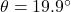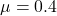Distance,d=5.02 m

a.Work done=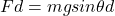Where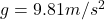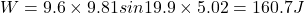b.Increase in internal energy of the crate,E=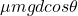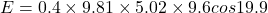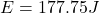c.Work done by 102 N=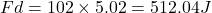d.Change in kinetic energy=-160.7+512.04-177.75=173.6 J

e.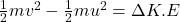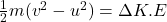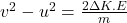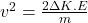$$+u^2$$